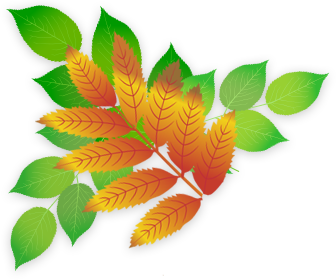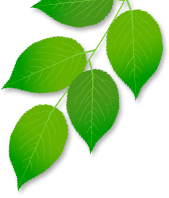## Year 3 Numeracy

In Year 3 children are taught to read and write numbers up to 1000 and are expected to learn the 3,4 and 8 times tables. They are shown how to use more formal written methods to add and subtract numbers with three digits and perform increasingly complex mental arithmetic with two digit numbers. They multiply and divide numbers using informal written methods. Their understanding of fractions is extended to include tenths and they are shown how to read and record the time to the nearest minute. They learn that angles are a unit of turn and learn about right angles.

These are the objectives that children will be taught in Numeracy during Year 3.

Year 3: Number and Place Value

• count from 0 in multiples of 4, 8, 50 and 100; find 10 or 100 more or less than a given number
• recognise the place value of each digit in a three-digit number (hundreds, tens, ones)
• compare and order numbers up to 1000
• identify, represent and estimate numbers using different representations
• read and write numbers up to 1000 in numerals and in words
• solve number problems and practical problems involving these ideas.

• add and subtract numbers mentally, including:
• add and subtract numbers with up to three digits, using formal written methods of columnar addition and subtraction
• estimate the answer to a calculation and use inverse operations to check answers
• solve problems, including missing number problems, using number facts, place value, and more complex addition and subtraction.

Year 3: Multiplication and Division

• recall and use multiplication and division facts for the 3, 4 and 8 multiplication tables
• write and calculate mathematical statements for multiplication and division using the multiplication tables that they know, including for two-digit numbers times one-digit numbers, using mental and progressing to formal written methods
• solve problems, including missing number problems, involving multiplication and division, including positive integer scaling problems and correspondence problems in which n objects are connected to m objects.

Year 3: Fractions (including decimals and percentages)

• count up and down in tenths; recognise that tenths arise from dividing an object into 10 equal parts and in dividing one-digit numbers or quantities by 10
• recognise, find and write fractions of a discrete set of objects: unit fractions and non-unit fractions with small denominators
• recognise and use fractions as numbers: unit fractions and non-unit fractions with small denominators
• recognise and show, using diagrams, equivalent fractions with small denominators
• add and subtract fractions with the same denominator within one whole [for example, 5⁄7 + 1⁄7 = 6⁄7 ]
• compare and order unit fractions, and fractions with the same denominator
• solve problems that involve all of the above.

Year 3: Measurement

• measure, compare, add and subtract: lengths (m/cm/mm); mass (kg/g); volume/capacity (l/ml)
• measure the perimeter of simple 2-D shapes
• add and subtract amounts of money to give change, using both £ and p in practical contexts
• tell and write the time from an analogue clock, including using Roman numerals from I to XII, and 12-hour and 24-hour clocks
• estimate and read time with increasing accuracy to the nearest minute; record and compare time in terms of seconds, minutes and hours; use vocabulary such as o’clock, a.m./p.m., morning, afternoon, noon and midnight
• know the number of seconds in a minute and the number of days in each month, year and leap year
• compare durations of events [for example to calculate the time taken by particular events or tasks].

Year 3: Geometry - properties of shapes

• draw 2-D shapes and make 3-D shapes using modelling materials; recognise 3-D shapes in different orientations and describe them
• recognise angles as a property of shape or a description of a turn
• identify right angles, recognise that two right angles make a half-turn, three make three quarters of a turn and four a complete turn; identify whether angles are greater than or less than a right angle
• identify horizontal and vertical lines and pairs of perpendicular and parallel lines.

Year 3: Statistics

• interpret and present data using bar charts, pictograms and tables
• solve one-step and two-step questions [for example, ‘How many more?’ and ‘How many fewer?’] using information presented in scaled bar charts and pictograms and tables.